Courses

# Test: Distance Formula 3D Geometry

## 10 Questions MCQ Test Mathematics (Maths) Class 11 | Test: Distance Formula 3D Geometry

Description
This mock test of Test: Distance Formula 3D Geometry for JEE helps you for every JEE entrance exam. This contains 10 Multiple Choice Questions for JEE Test: Distance Formula 3D Geometry (mcq) to study with solutions a complete question bank. The solved questions answers in this Test: Distance Formula 3D Geometry quiz give you a good mix of easy questions and tough questions. JEE students definitely take this Test: Distance Formula 3D Geometry exercise for a better result in the exam. You can find other Test: Distance Formula 3D Geometry extra questions, long questions & short questions for JEE on EduRev as well by searching above.
QUESTION: 1

### The equation representing the set of points which are equidistant from the points (1, 2 , 3) and (3 , 2 , -1) is

Solution:

Let the given points be A (1, 2, 3) and B (3, 2, -1) Let P(x, y, z) be any point which is equidistant from the points A and B.
Then PA = PB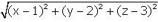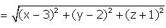⇒ (x - 1)2 + (y - 2)2 + (z - 3)2
= (x - 3)2 + (y - 2)2 + (z +1)2
⇒ x2 +1 - 2x + y2 + 4 - 4y + z2 + 9 - 6z
= x2 + 9 - 6x + y2 + 4 - 4y + z2 +1+2z
⇒ 6x - 2x - 4y + 4y -  6z - 2z = 0
⇒ 4x - 8z = 0
⇒ x - 2z = 0
This is the required equation of the set of points in reference.

QUESTION: 2

### The locus of the point which is equidistant from the points A(0, 2, 3) and B(2, -2, 1) is:

Solution: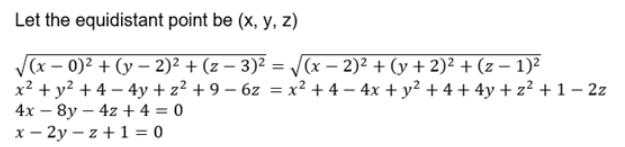QUESTION: 3

### The distances of the point (1, 2, 3) from the coordinate axes are A, B and C respectively. Which option is correct?

Solution:

Given points (1,2,3)
Pt A (1,0,0) on x axis
Pt B (0,2,0) on y axis
Pt C (0,0,3) on z axis
A2 = [0 + 2 + 3]
A2 = 13
B2 = [1 + 0 + 3]
B2 = 10
C2 = [1 + 2 + 0]
C2 = 5

a)2A2C2 = 13B2
⇒ 2(13)(5) = 13(10)
⇒ 130 = 130 {true}

b) A2 = 2C2
⇒ (13) = 2(5)
⇒ 13 = 10 {false}

c)B2 = 3C2
⇒ 10 = 3(5)
⇒ 10 = 15 {false}

d)A2 = B2 + C2
⇒ (13) = 10 + 5
⇒ 13 = 15 {false}

QUESTION: 4

A and B be the points (3, 4, 5) and (-1, -3, -7), respectively, the equation of the set of points P such that PA2 + PB2 = k2, where k is a constant will

Solution:
QUESTION: 5

A(4,7,8) B(2,3,4) , C (-1,-2,1) and D(1,2,5) are vertices of a quadrilateral. The quadrilateral is a

Solution:

AB =  [(2−4)2 +(3−2)+(4−8)2]1/2
AB=  [(−2)2 + (1)2 + (−4)2]^1/2
AB =  (21)1/2
Similarly you find that BC=  (43)1/2
CD= (33)1/2  and DA= (43)1/2
Hence opposite sides of quadrilateral are equal, Now we check the diagonals
AC=  [(-1-4)2 + (−2-7)2 + (1−8)2]1/2
AC=  (155)1/2
similarly BD=  (3)1/2
Diagonals are not equal
direction ratio of line passing through AB is (-2,-4,-4)
direction ratio of line passing through  CD is (2,4,4), As the dr of AB and CD are proportional which means AB is parallel to CD,
Similarly check for BC and DA then you will find that they are also parallel
Hence it is parallelogram.

QUESTION: 6

Find the points on z-axis which are at a distance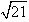from the point (1, 2, 3).

Solution:

Let the point on Z axis be given as (0,0,z).  The distance between (1,2,3) and (0,0,z) is given as [(1)2 + (2)2 + (3-z)2]½ = (21)1/2
5+(3−z)2=21
z2−6z−7=0
z=7,z = −1
Hence points are (0,0,7),(0,0,−1)

QUESTION: 7

The distance of the point (3, 4, 5) from X-axis is:

Solution:
QUESTION: 8

The distance between the points P(x1, y1, z1) and Q (x2, y2, z2) is given by:

Solution: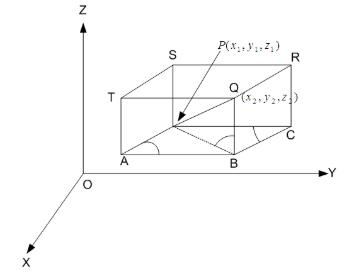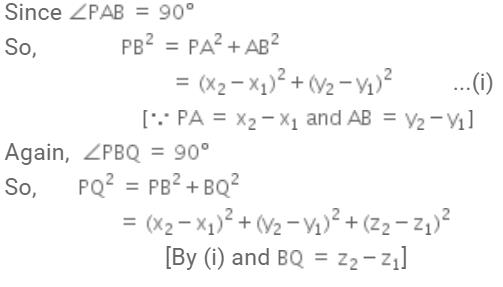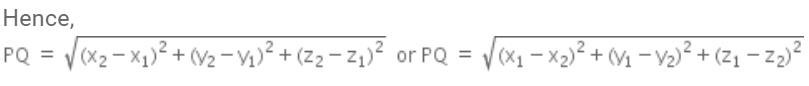QUESTION: 9

The points (1, -1, 3), (2, -4, 5) and (5, -13, 11) are:

Solution:
QUESTION: 10

If P,Q,R be the mid points of sides AB, AC and BC of triangle ABC. Where , A (0, 0, 6), B (0,4, 0)and (6, 0, 0).Then

Solution: